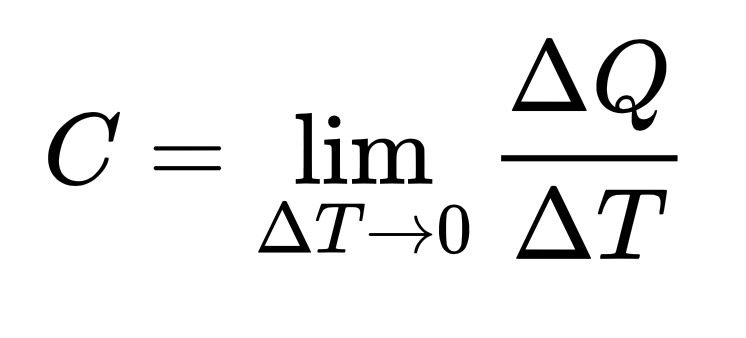A# heat capacityThe heat capacity of an object, C, is the limit as ΔT tends to zero of ΔQ over ΔT, where ΔQ is the amount of heat that must be added to the object (of mass M) in order to raise its temperature by ΔT.

Heat capacity, also called thermal capacity, is the amount of heat needed to raise the temperature of an object through 1°C, either at constant pressure or at constant volume and without inducing chemical changes or a change of phase. Numerically it is equal to the product of the mass of the object and its specific heat.

Heat capacity is measured in joules per kilogram (SI units) or calories per gram.